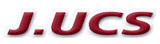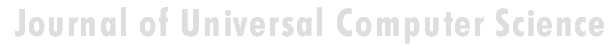Search
 Submission Procedure share: |available in:   PDF (283 kB) PS (105 kB)

get:
 Similar Docs BibTeX Write a comment
get:

DOI:   10.3217/jucs-006-09-0861

Uncertainty Propagation in Heterogeneous Algebras for Approximate Quantified Constraint Solving

Stefan Ratschan (Research Institute for Symbolic Computation, Austria)

Abstract: When trying to solve quantified constraints (i.e., first-order formulas over the real numbers) exactly, one faces the following problems: First, constants coming from measurements are often only approximately given. Second, solving such constraints is in general undecidable and for special cases highly complex. Third, exact solutions are often extremely complicated symbolic expressions. In this paper we study how to do approximate computation instead of working on approximate inputs and producing approximate output. For this we show how quantiffied constraints can be viewed as expressions in heterogeneous algebra and study how to do uncertainty propagation there. Since set theory is a very fundamental approach for representing uncertainty, also here we represent uncertainty by sets. Our considerations result in a general framework for approximate computation that can be applied in various different domains.

Keywords: computational logic, constraints, interval computation, uncertainty

Categories: F.4.1, I.2.3, I.2.4